Function Repository Resource:

# FindFirstNumberInstance

Find the first number that matches a certain requirement

Contributed by: Sander Huisman
 ResourceFunction["FindFirstNumberInstance"][f] finds the first integer instance from the range 1 to ∞ for which f returns True ResourceFunction["FindFirstNumberInstance"][f,max] finds the first integer instance from the range 1 to max for which f returns True ResourceFunction["FindFirstNumberInstance"][f,{min, max}] finds the first integer instance from the range min to max for which f returns True ResourceFunction["FindFirstNumberInstance"][f,{min,max,step}] finds the first instance from the range min to max in steps of step for which f returns True ResourceFunction["FindFirstNumberInstance"][f,spec, n] finds the first n instances for which f returns True

## Details

The third argument n can be set to ∞, and can return all the cases for the case that spec is a finite range.
Unlike in SelectFirst or FirstCase, no upper bound needs to be given.

## Examples

### Basic Examples (2)

Find the first prime starting from 1:

 In:=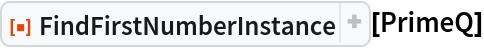Out=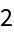Find the first 10 composite numbers:

 In:=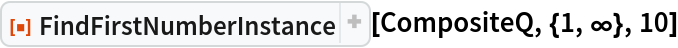Out=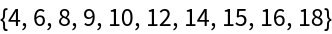### Scope (5)

Find the first 15 square numbers that are a multiple of 7:

 In:=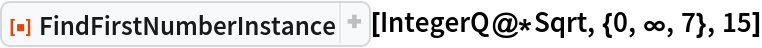Out=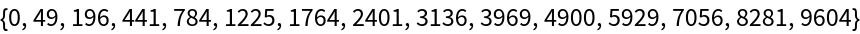Find the first 15 palindromic primes:

 In:=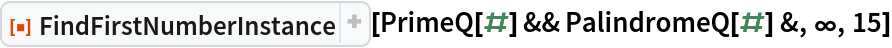Out=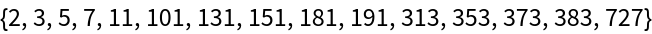Find the first 12 palindromes that are a multiple of 3:

 In:=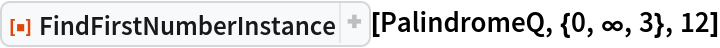Out=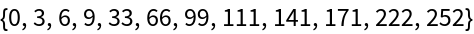Use an iterator with Real step size:

 In:=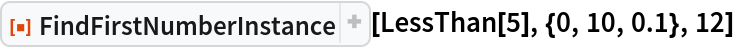Out=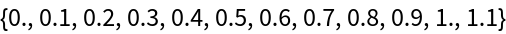The iterator can not only be numbers, it can also be a symbolic expression:

 In:=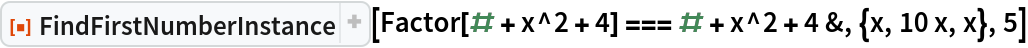Out=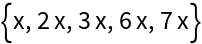### Possible Issues (2)

FindFirstInstance will return less instances if no more instances exist:

 In:=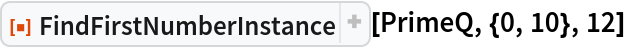Out=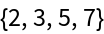For the case that there is no third argument and there is no match, FindFirstNumberInstance returns Missing:

 In:=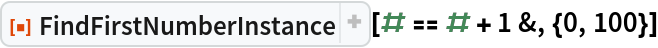Out=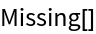An empty list is returned if there is a third argument:

 In:=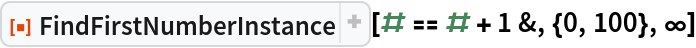Out=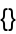### Neat Examples (2)

Find the first 12 palindromic composites that are a multiple of 7:

 In:=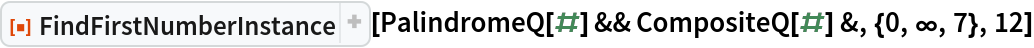Out=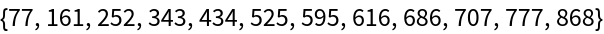Find the first 20 emirp primes:

 In:=Out=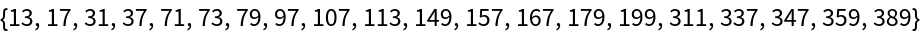SHuisman

## Version History

• 1.0.1 – 04 October 2022
• 1.0.0 – 23 September 2022